# Understanding Z-Scores in Statistics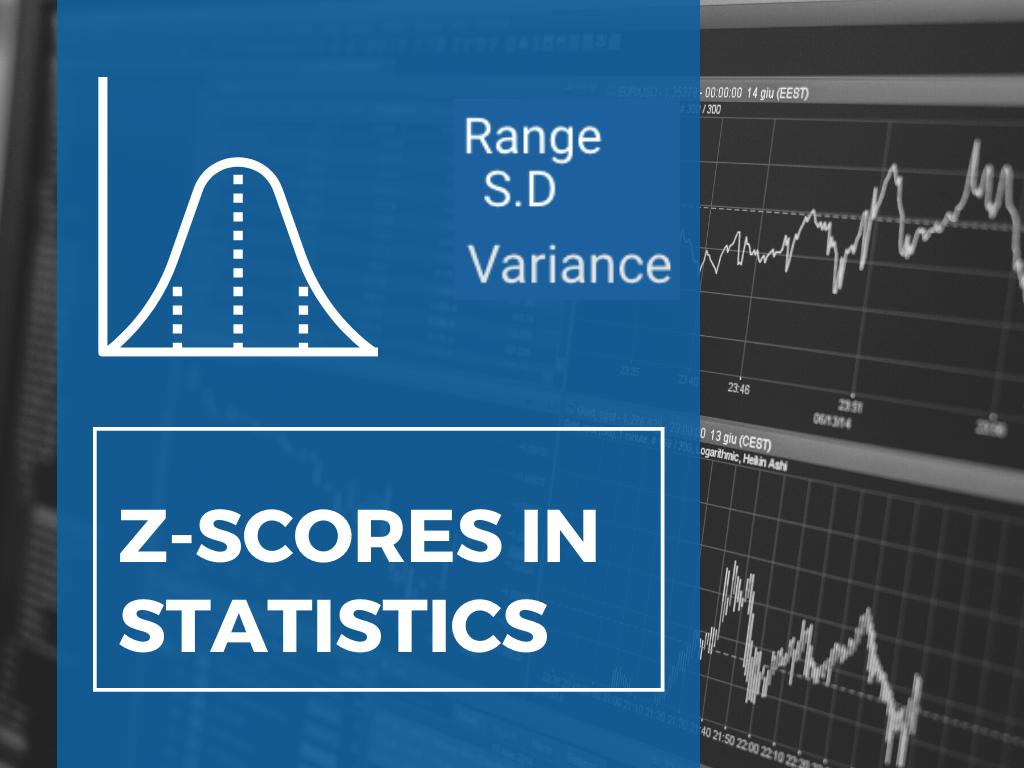Most of us have heard/used the terms mean, median, and mode in Statistics. To get a better idea, you can read my article on them here. Now, let us talk about measures of variability and Z-scores. How many of you know that Z-scores are used to estimate student’s academic records in Japan?

Most of us have heard/used the terms mean, median, and mode in Statistics. To get a better idea, you can read my article on them here. Now, let us talk about measures of variability and Z-scores. How many of you know that Z-scores are used to estimate student’s academic records in Japan? Z-scores are also used by WHO in child growth surveys. To understand Z-scores, let us try and understand the intuition behind measures of variability.

## Measures of Variability

Variability(also called as spread, dispersion) refers to how spread out the data is.

### Range

For Instance, Consider the following distributions —

A = [6, 6, 6, 6]

B = [1, 6, 1, 6]

The values in list A do not vary whereas values in list B show some variability. If we were to assign a variability score to both the lists we would assign** 0** to A. What would be the score of B?

We need a metric to describe the variability of a given distribution. One intuitive way would be to find the difference between the maximum and minimum value of the distribution(also known as the range). For List A, the maximum value and the minimum value is 6,

Hence, max(A) — min(A) = 0

For List B, the maximum value is 6and the minimum value is 1.

Hence, max(B) — min(B) = 5.

In general terms, the range of a distribution X is given by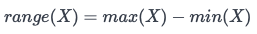The problem with the range is that it considers only 2 values from the distribution. For instance, consider the following distribution -

C = [1, 1, 1, 1, 1, 1, 1, 1, 1, 21]

Since there is not much variability in C, we would expect the variability to be close to zero. If we calculate the range of the above distribution

range(C) = max(C)-min(C) = 21–1 = 20.

This is very high when compared to zero and does not seem to be an appropriate measure for variability. Since the range considers only two values, it is extremely sensitive to outliers.

### Variance and Standard deviation

To get a more balanced measure of variability, it is better if we take into account each value of the distribution. One way to solve this would be -

1. Take a reference value (mean/median).

2. Find out the distance of each value from the reference value.

3. Find the mean of all distances. (Sum of distances / total number of distances)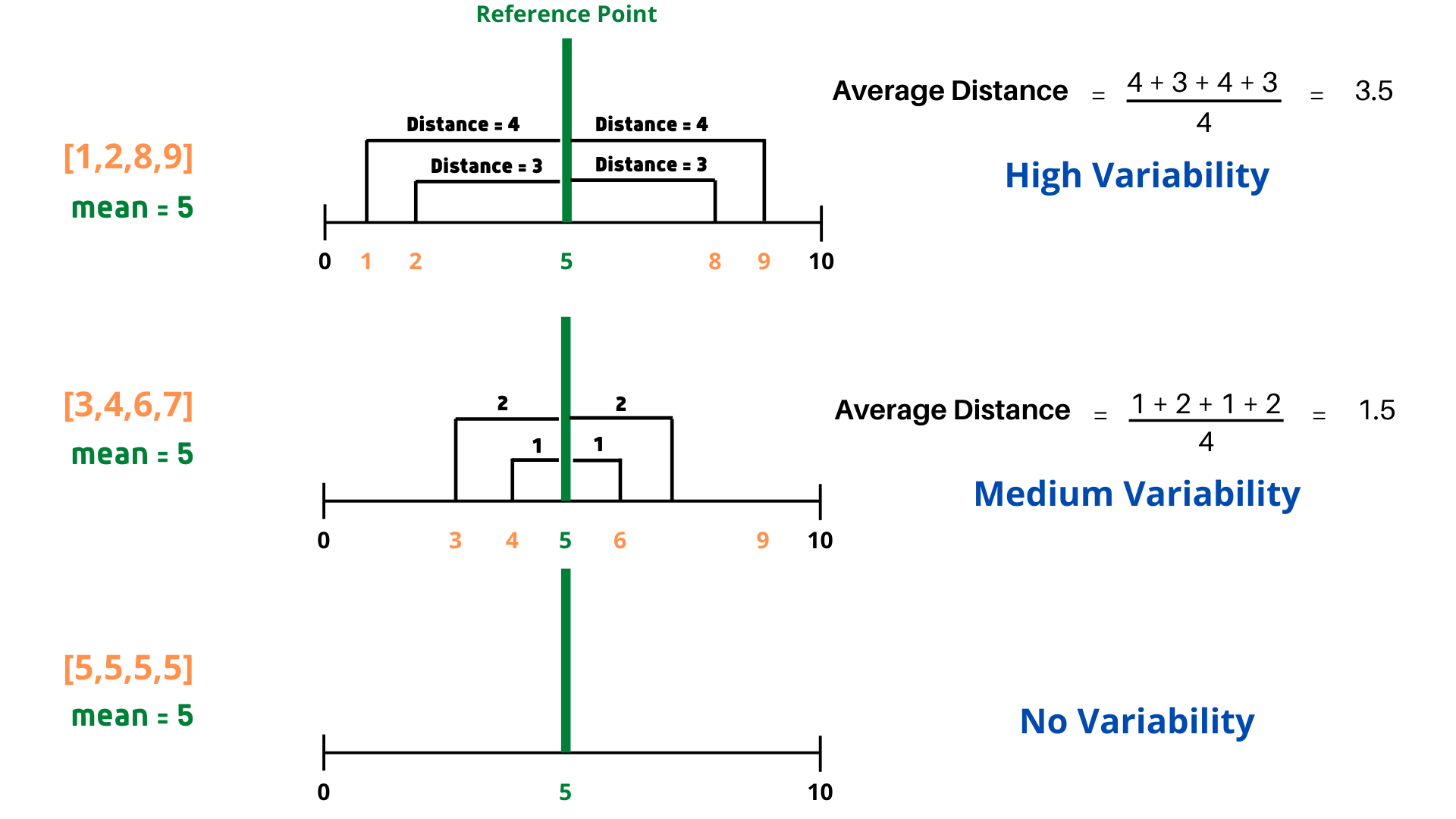Designed using Canva.

Algebraically this method can be formulated in the following way. Consider mean to be μ and distribution to be [X₁, X₂, X₃, X₄, …..Xn].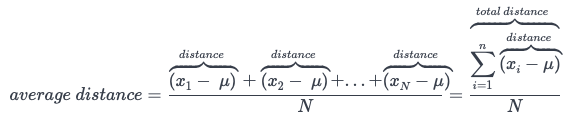Now, let us consider the previously used distribution -

C = [1, 1, 1, 1, 1, 1, 1, 1, 1, 21] , mean = 3

If we calculate the average distance, we observe that the numerator would be zero. To overcome this problem, we consider the square of distances instead of only differences in the numerator.

Even though taking absolute values is another solution, the square function is preferred because it has

a)better properties (like smooth and differentiable)

b)also magnifies the effect of outliers.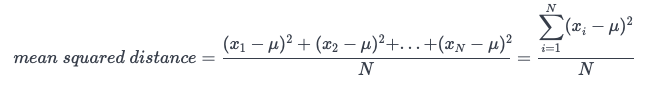This means squared distance is also known as a variance. Now let us calculate the variance for the distribution C.

The value of 36 is higher than expected. This is a result of directly squaring the distances and is being reflected in the higher values. And in certain instances, a case of units mismatch arises too.

To overcome the above problems, we take the square root of the sum of squared distances and this is known as standard deviation.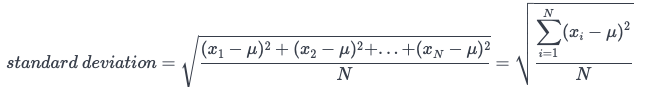In general, the standard deviation is most frequently used as a measure of spread. From now on, we will be working with a data set that describes the details of houses sold from 2016 to 2010 in the city of Ames (in America). There are 2930 rows in the data set, and each row describes a house.

Let us try and find out the standard deviation of the “SalesPrice” column in the houses dataset.

``````print(standard_deviation(houses['SalePrice']))

Output - 79873.05865192247``````

The mean of the “SalesPrice” column is

``````print(houses['SalePrice'].mean())

Output - 180796.0600682594``````

The mean tells us that the average price of a house is roughly \$1,80,796 but that does not imply all the houses will have a price of \$1,80,796. There will be houses that cost around \$1,20,000 and there will be few that may cost \$2,40,000 too. The standard deviation will give us a picture of how the prices vary with respect to the mean. So, an S.D of \$79873 means the prices roughly by \$79873 above and below the mean.

The standard deviation is not setting boundaries for the price limits but is trying to explain that majority of houses fall under the category of mean+S.D *or *mean-S.D.

## HTML entity encoder decoder Online

Online Data Science Training in Noida at CETPA, best institute in India for Data Science Online Course and Certification. Call now at 9911417779 to avail 50% discount.

## 50 Data Science Jobs That Opened Just Last Week

Data Science and Analytics market evolves to adapt to the constantly changing economic and business environments. Our latest survey report suggests that as the overall Data Science and Analytics market evolves to adapt to the constantly changing economic and business environments, data scientists and AI practitioners should be aware of the skills and tools that the broader community is working on. A good grip in these skills will further help data science enthusiasts to get the best jobs that various industries in their data science functions are offering.

## Statistics for Data Science

Statistics for Data Science and Machine Learning Engineer. I’ll try to teach you just enough to be dangerous, and pique your interest just enough that you’ll go off and learn more.

## Data Science With Python Training | Python Data Science Course | Intellipaat

🔵 Intellipaat Data Science with Python course: https://intellipaat.com/python-for-data-science-training/In this Data Science With Python Training video, you...

## AI or Data Science? | Artificial Intelligence And Data Science Career

There are many intersections and overlaps between AI and data science. AI has numerous subsets, like Machine Learning (ML), Deep Learning (DL), and Natural Language Processing (NLP). With many career opportunities in both fields, there are lots of conflicting perspectives on educational paths for starting a career in one of these fields.